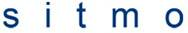# Angular momentum quantum number and angle

## Homework Statement

What are the possible values for the magnetic quantum number ml for an electron whose orbital quantum number is l = 4? What are the allowed values of the angles between L and the zaxis in this case?

## Homework Equations## The Attempt at a Solution

Is Ml equal to four for the first part? Because the angular momentum quantum number is equal to four?

I have no idea for the second part. I don't have the gradient of the magnetic field of the z-component and don't have any distance or velocity values.

gabbagabbahey
Homework Helper
Gold Member

## Homework EquationsHow exactly is this equation relevant?The problem is in regard to an electron orbiting a nucleus, not an electron moving through an inhomogeneous magnetic field as in the Stern-Gerlach experiment.

Is Ml equal to four for the first part? Because the angular momentum quantum number is equal to four?

Open your textbook up and read the section on hydrogen like atoms and angular momentum. The allowed values of $m_l$ for any given value of $l$ will be clearly stated in your text.

I have no idea for the second part.

If $\theta$ is the angle between $\mathbf{L}$ and the z-axis, then $L_z\equiv \mathbf{L}\cdot \textbf{k}=|\mathbf{L}|\cos\theta[/tex]. So, in terms of operators, you would expect $$\hat{L_z}^2=\hat{L}^2\cos^2\hat{\theta}$$ Where the hat is to denote that we are talking about operators here. (i.e. $$\hat{\theta}$$ is an operator whose value upon measurement corresponds to the angle between [itex]\mathbf{L}$ and the z-axis)

What are the allowed values when you measure [tex]\hat{L_z}^2[/itex] for an electron in the state $l=4$?

Last edited: